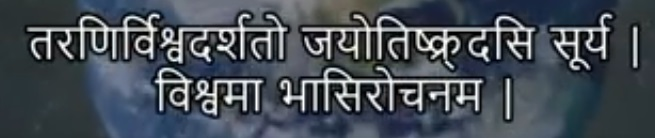Speed of Light: Rigveda vs Modern ScienceThe above verse is given from verse number 50 from Rigveda book number 1 and its literal translation is given as follows

Light travels 2202 yojanas in one-half nimisha

So let us discuss this in detail

Nimisha is a unit of time used ancient India which is having the following conversions

1 kastha = 15 nimishas

1 kala = 30 kasthas

1 mahurta = 30.3 kalas

1 diva ratri = 30 mahurtas

and

1 diva ratri = 1 day – night = 24 hours of modern time unit

if you do a little bit of maths you can see that it comes to

1 nimisha = 0.2112 seconds of modern time

so one-half nimisha = 0.1056 seconds of modern time

coming to Yojan

1 yojan = 9.09 miles

so going by the formula that distance = speed * time

we get the speed of light as

189547 miles per hour

or in kilometers as

3.05 * 10^8 meters per second  = 305000000 = 3 lac km per sec

as per the modern day calculations the speed of light is also 3 lac kilometers per second

299 792 458 meters per second = 3 lac km per sec

So we can see that the modern day science has the same results as was written in rigvedas

Paid Horoscope Analysis

Dear friends please pay our fee by going to this link and then fill the horoscope form